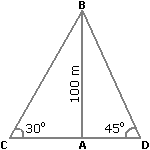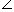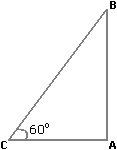# Basic Numeracy (Test 1)

## Upsc Civil Services Entrance Exams : General Studies Test

| Home | | Upsc Civil Services Entrance Exams | | General Studies Test | | Basic Numeracy |Basic Numeracy
| Basic Numeracy |
Q.1
A train 125 m long passes a man, running at 5 km/hr in the same direction in which the train is going, in 10 seconds. The speed of the train is:
A. 45 km/hr
B. 50 km/hr
C. 54 km/hr
D. 55 km/hr
Explaination / Solution:

 Speed of the train relative to man =125m/sec 10

 =25m/sec. 2

 =25 x 18km/hr 2 5

= 45 km/hr.

Let the speed of the train be x km/hr. Then, relative speed = (x - 5) km/hr.x - 5 = 45x = 50 km/hr.

Workspace
Report
Q.2
Two ships are sailing in the sea on the two sides of a lighthouse. The angle of elevation of the top of the lighthouse is observed from the ships are 30° and 45° respectively. If the lighthouse is 100 m high, the distance between the two ships is:
A. 173 m
B. 200 m
C. 273 m
D. 300 m
Explaination / Solution:

Let AB be the lighthouse and C and D be the positions of the ships.Then, AB = 100 m,ACB = 30° andADB = 45°.

 AB = tan 30° = 1AC = AB x √3 = 100√3 m. AC √3

 AB = tan 45° = 1AD = AB = 100 m. ADCD = (AC + AD) = (100√3 + 100) m = 100(√3 + 1) = (100 x 2.73) m = 273 m.

Workspace
Report
Q.3
The angle of elevation of a ladder leaning against a wall is 60º and the foot of the ladder is 4.6 m away from the wall. The length of the ladder is:
A. 2.3 m
B. 4.6 m
C. 7.8 m
D. 9.2 m
Explaination / Solution:

Let AB be the wall and BC be the ladder.Then,ACB = 60º and AC = 4.6 m.

 AC = cos 60º = 1 BC 2BC = 2 x AC = (2 x 4.6) m = 9.2 m.

Workspace
Report
Q.4
A sum of money at simple interest amounts to Rs. 815 in 3 years and to Rs. 854 in 4 years. The sum is:
A. Rs. 650
B. Rs. 690
C. Rs. 698
D. Rs. 700
Explaination / Solution:

S.I. for 1 year = Rs. (854 - 815) = Rs. 39.

S.I. for 3 years = Rs.(39 x 3) = Rs. 117.Principal = Rs. (815 - 117) = Rs. 698.

Workspace
Report
Q.5
Mr. Thomas invested an amount of Rs. 13,900 divided in two different schemes A and B at the simple interest rate of 14% p.a. and 11% p.a. respectively. If the total amount of simple interest earned in 2 years be Rs. 3508, what was the amount invested in Scheme B?
A. Rs. 6400
B. Rs. 6500
C. Rs. 7200
D. Rs. 7500
E. None of these
Explaination / Solution:

Let the sum invested in Scheme A be Rs. x and that in Scheme B be Rs. (13900 - x).

 Then,x x 14 x 2+(13900 - x) x 11 x 2= 3508 100 10028x - 22x = 350800 - (13900 x 22)6x = 45000x = 7500.

So, sum invested in Scheme B = Rs. (13900 - 7500) = Rs. 6400.

Workspace
Report
Q.6
The cost price of 20 articles is the same as the selling price of x articles. If the profit is 25%, then the value of x is:
A. 15
B. 16
C. 18
D. 25
Explaination / Solution:

Let C.P. of each article be Re. 1 C.P. of x articles = Rs. x.

S.P. of x articles = Rs. 20.

Profit = Rs. (20 - x).20 - x x 100 = 25x2000 - 100x = 25x

125x = 2000x = 16.

Workspace
Report
Q.7
In a certain store, the profit is 320% of the cost. If the cost increases by 25% but the selling price remains constant, approximately what percentage of the selling price is the profit?
A. 30%
B. 70%
C. 100%
D. 250%
Explaination / Solution:

Let C.P.= Rs. 100. Then, Profit = Rs. 320, S.P. = Rs. 420.

New C.P. = 125% of Rs. 100 = Rs. 125

New S.P. = Rs. 420.

Profit = Rs. (420 - 125) = Rs. 295.Required percentage =295 x 100% = 1475 % = 70% (approximately). 420 21

Workspace
Report
Q.8
Two students appeared at an examination. One of them secured 9 marks more than the other and his marks was 56% of the sum of their marks. The marks obtained by them are:
A. 39, 30
B. 41, 32
C. 42, 33
D. 43, 34
Explaination / Solution:

Let their marks be (x + 9) and x.

 Then, x + 9 = 56 (x + 9 + x) 10025(x + 9) = 14(2x + 9)3x = 99x = 33

So, their marks are 42 and 33.

Workspace
Report
Q.9
A fruit seller had some apples. He sells 40% apples and still has 420 apples. Originally, he had:
A. 588 apples
B. 600 apples
C. 672 apples
D. 700 apples
Explaination / Solution:

Suppose originally he had x apples.

Then, (100 - 40)% of x = 420.60 x x = 420 100x =420 x 100= 700. 60

Workspace
Report
Q.10
It was Sunday on Jan 1, 2006. What was the day of the week Jan 1, 2010?
A. Sunday
B. Saturday
C. Friday
D. Wednesday
Explaination / Solution:

On 31st December, 2005 it was Saturday.

Number of odd days from the year 2006 to the year 2009 = (1 + 1 + 2 + 1) = 5 days.On 31st December 2009, it was Thursday.

Thus, on 1st Jan, 2010 it is Friday.

Workspace
Report